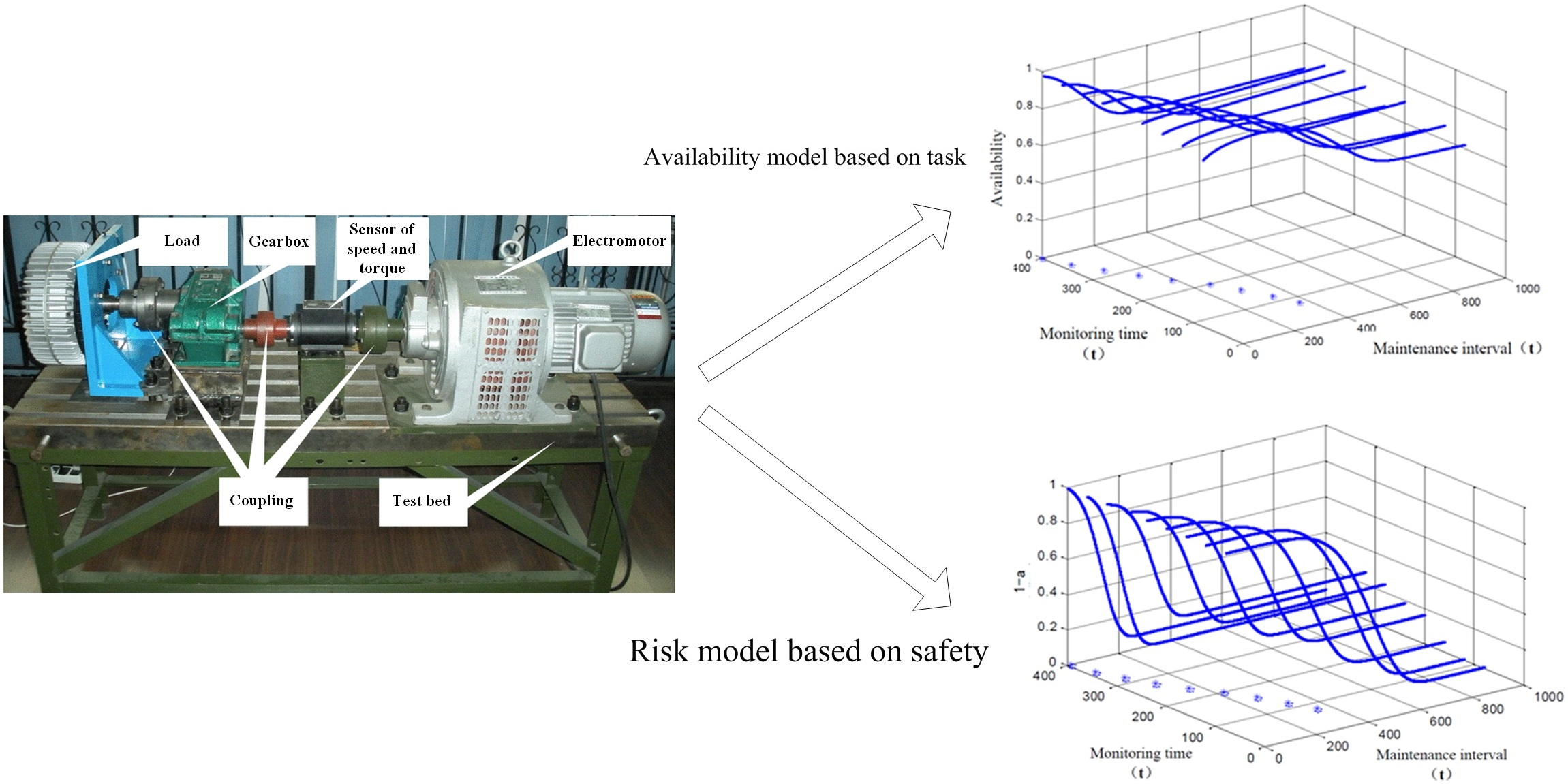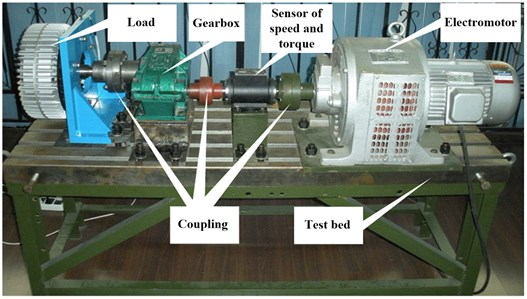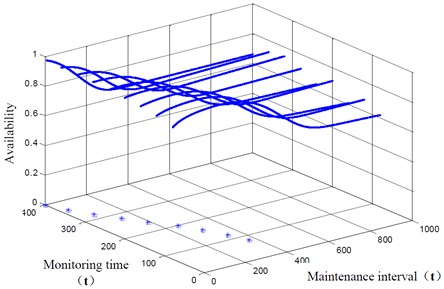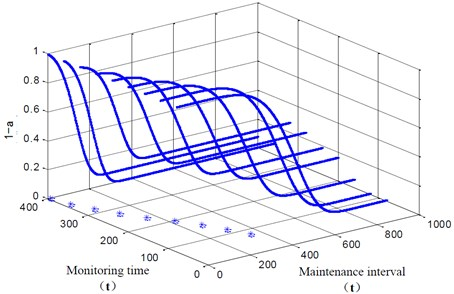Vibroengineering PROCEDIA

Published: 25 March 2021

# Availability model based on task and risk model based on safety for condition based maintenance

Menghan Li1
Xin Liu2
Kun Yang3
Zhenguo Li4
1, 4National Engineering Laboratory for Mobile Source Emission Control Technology, China Automotive Technology and Research Center Co., Ltd., Tianjin, China
2, 3Institute of Medical Support Technology, Academy of Systems Engineering, Academy of Military Science of PLA, Tianjin, China
Corresponding Author:
Xin Liu
Views 28

#### Abstract

The most important thing for condition based maintenance (CBM) is the maintenance decision-making, while the existing research mainly considers system maintenance decision-making from the economic point of view, but the actual situation is that the availability and safety of the system are more important in most cases. In this paper, the maintenance decision-making model of the system is established by giving priority to the availability and safety of the system, which is more in line with the engineering practice and lays a foundation for the application of condition based maintenance.#### Highlights

• The availability model based on task for condition based maintenance is proposed
• The risk model based on safety for condition based maintenance is proposed
• The model is useful to improve the availability of maintenance decision

## 1. Introduction

Nowadays, condition based maintenance (CBM) of multi-component systems optimization is studied [1-4], and the relationship between the main popular maintenance modes is analyzed . Many new methods are proposed, such as a new maintenance decision-making which is made by an artificial neural network, expert system and emulation technology is proposed to decrease the life cost . The maintenance decision-making optimization is proposed considering the influence of human reliability to minimize the cost . The discounted cost associated with a maintenance policy combining both condition-based and age-based criteria for preventive maintenance is proposed which provides a more realistic basis for optimizing the maintenance policies . A cost-based selective maintenance decision-making method was presented considering the influence of machine failure intensity .

From the above description, we can know that the maintenance decision-making model is becoming more and more abounding and practical. However, the existing models are focusing on the cost, ignoring the availability model and risk model which are play a more important role in the maintenance decision-making. Therefore, we proposed the availability model based on task and risk model based on safety for condition based maintenance in this paper in order to obtain the maximum availability and expected risk for system.

The rest of this paper is organized as follows. In Section 2 the availability model based on task and risk model based on safety for condition based maintenance is proposed. In Section 3, an experiment of gearbox is introduced. Then, the proposed method is applied to determine the gearbox optimal maintenance interval, which shows that different intervals will be obtained via the two models. Finally, the conclusions of the paper are drawn in Section 4.

## 2. Availability model and risk model

In actual engineering risk model based on safety is taken into account firstly to ensure the safety and the success of task. Secondly, availability model based on task is considered. In this paper, the components are assumed as well as new after maintenance. Moreover, maintenance decision-making model is proposed to determine the optimal maintenance interval.

### 2.1. Availability model based on task

In some cases, we can not only consider component economic efficiency, but also pay more attention to the task indicators. For example, we are more focused on availability and reliability of the aircraft, artillery and firearms in the battlefield, rather than how to reduce the cost. The economic factors will be considered only on the premise of ensuring availability. Then, the availability model based on task is needed to be established.

In an update cycle, the working time is consisted of as follows:

1
$E\left({T}_{w}\right)={t}_{i}+{T}_{i}\cdot p\left({x}_{i}\ge {T}_{i}|{Y}_{i}\right)+{\int }_{0}^{{T}_{i}}{x}_{i}\cdot p\left({x}_{i}|{Y}_{i}\right)d{x}_{i},$

where ${T}_{i}$ is optimal maintenance interval and ${t}_{i}$ is the $i$th monitoring time of system.

The time definition for a maintenance cycle is shown as follows:

2
$E\left(T\right)={t}_{i}+\left({T}_{i}+{T}_{p}\right)\cdot p\left({x}_{i}\ge {T}_{i}|{Y}_{i}\right)+{\int }_{0}^{{T}_{i}}\left({x}_{i}+{T}_{f}\right)p\left({x}_{i}|{Y}_{i}\right)d{x}_{i},$

where ${T}_{p}$ is an average maintenance interval and ${T}_{f}$ is average time for corrective maintenance.

The availability model is obtained as follows:

3
$A\left({T}_{i}\right)=\frac{E\left({T}_{w}\right)}{E\left(T\right)}=\frac{{t}_{i}+{T}_{i}\cdot p\left({x}_{i}\ge {T}_{i}|{Y}_{i}\right)+{\int }_{0}^{{T}_{i}}{x}_{i}\cdot p\left({x}_{i}|{Y}_{i}\right)d{x}_{i}}{{t}_{i}+\left({T}_{i}+{T}_{p}\right)\cdot p\left({x}_{i}\ge {T}_{i}|{Y}_{i}\right)+{\int }_{0}^{{T}_{i}}\left({x}_{i}+{T}_{f}\right)p\left({x}_{i}|{Y}_{i}\right)d{x}_{i}}.$

### 2.2. Risk model based on safety

In practice, the safety of the component is the most important. The purpose of maintenance decision-making is to realize accurate, timely and effective maintenance with decreasing maintenance costs at the same time. However, it does not mean that safety can be ignored. Once the safety problems occur, it may threaten the life safety of operating personnel or cause serious los. As a result, risk model based on safety need to be considered firstly.

The reliability or failure probability can be selected as the objective function in risk model based on safety. The optimal maintenance interval is determined through lower the fault probability of component to a pre-determined level. Assuming that component risk $\alpha$, the failure rate cannot be higher than the risk $\alpha$ in every monitoring process. Therefore, the probability of component not failure is:

4
$p\left({x}_{i}\ge {T}_{i}|{Y}_{i}\right)>1-\alpha .$

It can be described via the remaining useful life:

5
$1-{\int }_{0}^{{T}_{i}}p\left({x}_{i}|{Y}_{i}\right)d{x}_{i}>1-\alpha .$

The maintenance interval of risk model based on safety can be obtained by Eq. (5) when the remaining useful life has been known.

The maintenance decision-making usually takes reliability or risk rate as the objective function under the security requirement. So, the failure probability reduced to an acceptable level is used to determine the optimal maintenance interval.

## 3. Application

### 3.1. The introduction of experiment

In the experiment, the gear box was driven by a electromotor, and a load was added to simulate the real working state of the gearbox. The gearbox is shown in Fig. 1, and test results are shown in Table 1.

Fig. 1The experiment of gearboxTable 1The test results

 Monitoring time 80 120 160 200 240 280 320 360 400 ${x}_{i}\left(h\right)$ 361 321 281 241 201 161 121 81 41 Predicted RUL ($h$) 434 382 305 268 210 182 144 127 60

### 3.2. The results analysis

In the experiment, we assume that ${T}_{p}=$ 5 hours, ${T}_{f}=$ 15 hours and the risk is $\alpha =$ 0.05. The results of availability model based on task is shown in Fig. 2.

Fig. 2The results of availability model based on taskTable 2The results of availability model based on task

 Monitoring point $i$ 3 4 5 6 7 8 9 10 11 ${A}_{i}$ 0.963 0.9634 0.9638 0.9647 0.9647 0.9665 0.9697 0.973 0.9756 ${x}_{i}$ 402 362 322 282 242 202 162 122 82 ${T}_{i}$ 370 343 298 256 189 140 82 34 Repair immediately Prediction of RUL 485 423 367 257 207 231 139 151 53

The results of availability model based on task is shown in Table 2, which we can determine the maintenance interval. When maintenance interval that determined by availability model is earlier than RUL, the decision for system is obtained via availability model. On the other hand, when maintenance interval that determined by availability model is later than RUL, maintenance decision-making is obtained based on RUL. As we can see from Table 2, maintenance interval is earlier than RUL at all time, so optimal maintenance interval for maintenance decision is all based on availability model.

In the risk model based on safety, we assume that risk 0.05. Then, the result of maintenance decision-making based on risk model can be obtained which is shown in Fig. 3. As we can see from the figure, the safety index is higher with the decrease of the maintenance interval, which is consistent with our subjective experience. When $1-\alpha >$ 0.95 is satisfied, we will get the most optimal maintenance interval.

Fig. 3The results of risk model based on safetyThe results of risk model based on safety is shown in Table 3, which we can determine the maintenance interval. As we can see, the results based on risk model is earlier than the RUL of the gearbox, so it is necessary to propose maintenance decision based on risk model.

Table 3The results of risk model based on safety

 Monitoring point $i$ 3 4 5 6 7 8 9 10 11 ${x}_{i}$ 402 362 322 282 242 202 162 122 82 ${T}_{i}$ 353 316 274 221 165 114 69 34 16 Prediction of RUL 485 423 367 257 207 231 139 151 53

## 4. Conclusions

In actual engineering risk model based on safety is taken into account firstly to ensure the safety and the success of task. Secondly, availability model based on task is considered. In this paper, the availability model based on task and risk model based on safety for condition based maintenance is proposed, and the effectiveness of the method is verified by an experiment of gearbox. The availability model based on task and risk model based on safety is more in line with the actual situation of the project and can effectively improve the availability of maintenance decision.

#### Acknowledgements

The research is supported by National Engineering Laboratory for Mobile Source Emission Control Technology (No. NELMS2018C01), Science and Technology Directorate Project of Tianjin City (No. 18ZXSZSF00060) and the Natural Science Foundation of Hebei (No. E2019202198). The authors are grateful to all the reviewers and the editor for their valuable comments.